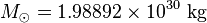# Solar mass facts for kids

Kids Encyclopedia Facts

The solar mass is a unit of measurement of mass. It is equal to the mass of the Sun, about 332,950 times the mass of the Earth, or 1,048 times the mass of Jupiter. Masses of other stars and groups of stars are listed in terms of solar masses. Its mathematical symbol and value are:$M_{\odot}=1.98892\times10^{30}\hbox{ kg}$ (kg being kilograms)Solar mass Facts for Kids. Kiddle Encyclopedia.